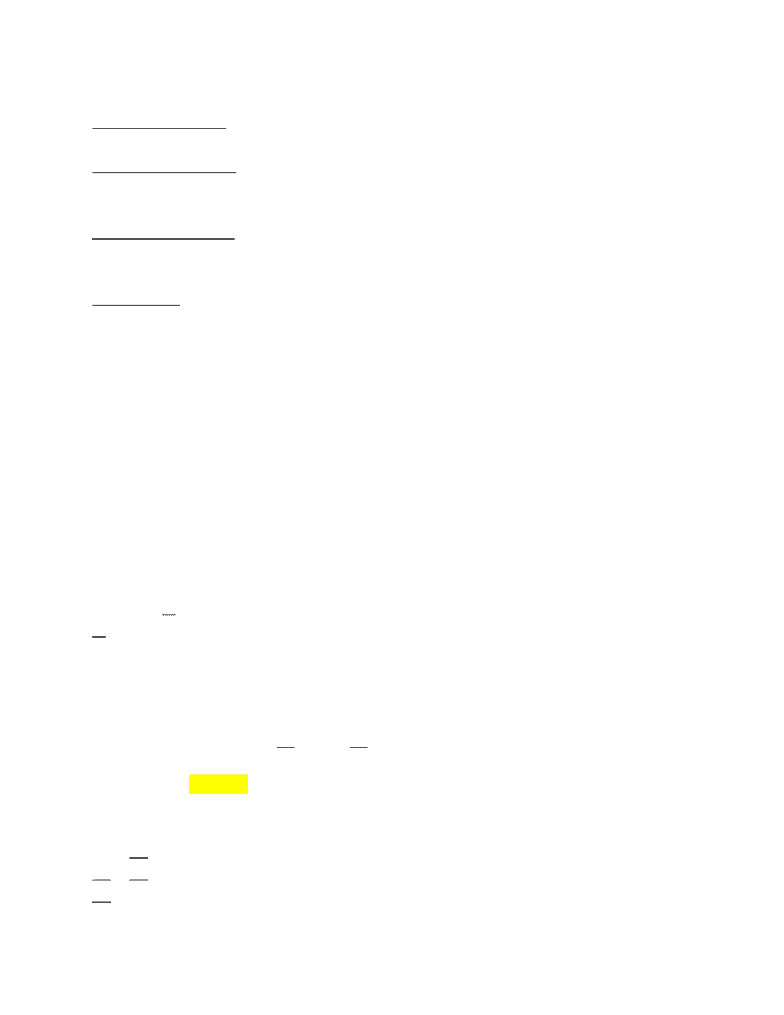# CSE 130 Lecture Notes - Lecture 8: Function Application, Kolmogorov Space, Length Function

16 views3 pagesGenerating Constraints
Lambda Abstraction (λx.e)
t0 = t1 → t2
Function declaration (f x = e)
t0 = t1 → t2
Function application (f x) @
t0 = t1 → t2 f x
Conditionals: (if x then y else z) :: t0 if
t1 = Bool t0
t0 = t2 x y z
t0 = t2 t0 t1 t2
f x = 2 + x = (+) 2 x = ((+) 2) x Fun
t0 = t1 → t6 f x @
t2 = t3 -> t4 t0 t1 t6
t4 = t1 → t6 @ x
t2 = Int -> Int -> Int t4 t1
t3 = Int + 2
t2 t3
Solve the Equations
t0 = t1 → t6
t2 = t3 → t4 t2 = t3 → (t1 → t6) t2 = Int->(t1 → t6)
t4 = t1 → t6
t2 = Int -> Int -> Int t3 → t4 = Int -> Int -> Int Int → t4 = Int->Int->Int
t3 = Int t3 → (t1 → t6) = Int->Int->Int
t1 → t6 = Int->Int
t1 = Int, t6 = Int
Int -> t4 = Int->(Int->Int)
t4 = Int->Int
t0 = t1 → t6 = Int->Int
Ex:
T0 = T1 → T4 T0 = (T3 → T4) → T4
T1 = T3 → T4 T0 = (Int->T4) → T4
T3 = Int f :: (Int->T4)->T4
Unlock document

This preview shows page 1 of the document.
Unlock all 3 pages and 3 million more documents.